# Motor Data

This dialog box includes the following areas and tabs:

See Common Tabs for information on the Location, Comments, Hyperlinks, or Collected Data tabs.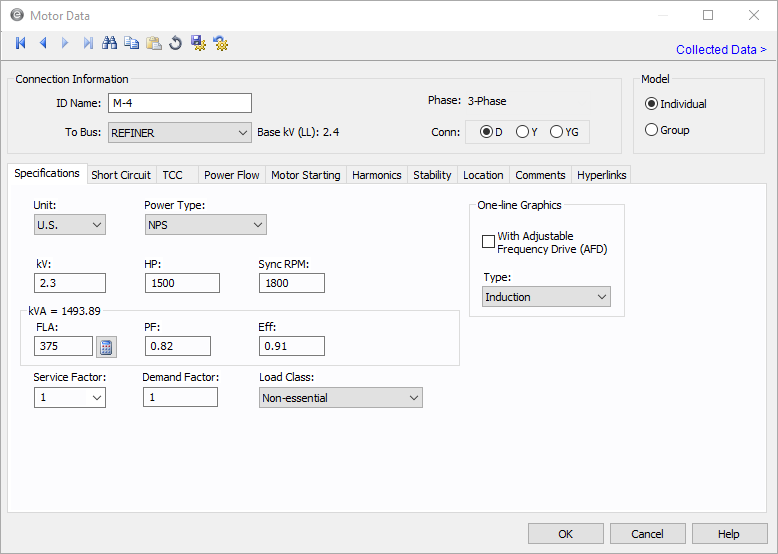Figure 1: Motor Data Dialog Box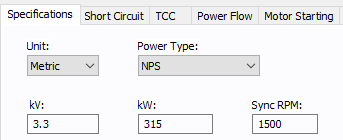Figure 2: Motor Data Dialog Box (Metric)

## Connection Information

Option Description
ID Name

Uniquely identifies the equipment item. The program automatically assigns a name, but you can change it, if needed. The name can be up to 16 characters long.

For motors, the program automatically assigns the names M-1, M-2, M-3, and so on.

To Bus

This is the ID name of the bus to which the equipment is connected. The base kV of the bus is indicated on the right.

Ensure that the To Bus has approximately the same base kV as the motor.

Phase

The phase of the item. Currently, this is for reference only.

Conn

The type of connection, which could be D (delta), Y (wye), or YG (wye-grounded). The default is D.

Model
• Individual: Labels the motor as an individual motor.
• Group: Labels the motor as a group of motors.

## Specifications Tab

Option Description
Unit Choose either US or Metric.
Power Type

The power type for the motor. Select from:

• NPS (normal power supply—for example, one that has no power backup)
• EPS (emergency power supply—for example, one that uses a generator for backup)
• CPS (critical power supply—for example, one that uses an uninterrupted power source)
kV Motor rated kV. This is normally 460 volts on a 480 volt base, or 2300 volts on a 2400 volt base.
Hp

Motor horsepower. Motors may be represented individually or as a lumped group. For a grouped motor, enter the total horsepower for the group.

This displays if the unit selected is US.

kW

Motor kilowatts. Motors may be represented individually or as a lumped group. For a grouped motor, enter the total kilowatts for the group.

This is displayed if the unit selected is metric.

RPM

Revolutions per minute. Used in output reports and defining equipment for ANSI or IEC standard short circuit calculation multipliers.

By default, ANSI uses 1800 and IEC uses 1500, unless the default equipment settings for motors are set to use a different value.

Load Class To specify the class in terms of importance. You can select Essential, Critical, or Non-essential. This field does not affect analysis. It can be used in database query to distinguish a certain load class from others. See Advanced Query for more information.
FLA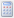Calculate looks up full load amps from NEC tables if the motor HP is listed in an NEC table. The full load amps field is not required, but a value greater than 0 affects how kVA is calculated. See Motor kVA Calculations.
PF Motor operating power factor. This is used with the Efficiency field to determine kVA. Motor groups should use an average value of power factor.
Eff Motor operating efficiency. This is used with the Power Factor field to determine kVA. Motor groups should use an average value of efficiency.
Service Factor The factor by which the motor can be safely overloaded. You can select any value between 1.0 and 1.25.
Demand Factor This factor is used to calculate the Demand kVA or Amps for the motor. The demand factor for a motor would be the ratio of maximum motor load to total rating of the motor.
One-line Graphics

Selecting this check box changes the motor symbol in the one-line as shown below.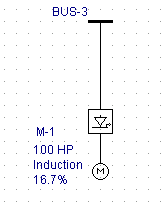Type

Motor type (induction, synchronous, synchronous condenser, or DC). This choice affects the motor impedances for short circuit calculations. The type also determines the one-line symbol for the motor.

These are the symbols if that are produced for each of the motor types if the connection type is D (delta) or Y (wye), which are both ungrounded types: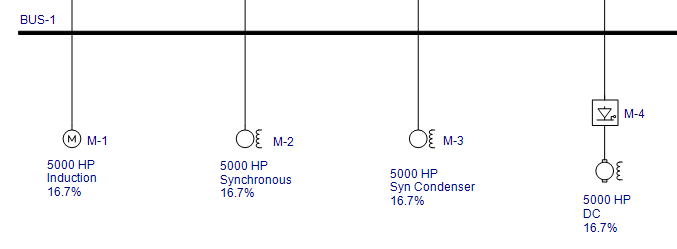These are the symbols that are produced for each of the motor types if the connection is YG (grounded wye):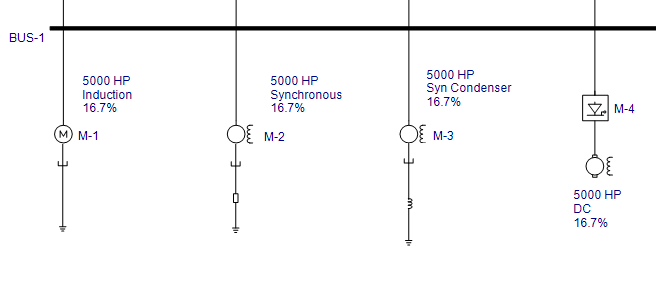### Motor kVA Calculations

There are two ways to calculate motor kVA in the Motor Specifications section of the motor dialog box. The one EasyPower uses depends on which fields you decide to enter. Below the kVA calculation methods are shown in priority order:

If the FLA is greater than 0, the motor kVA is determined by the following equation.

KVA = (1.73)(FLA)(kVmtr)

If the FLA is left blank or 0, the motor kVA will be determined from the following equation.

KVA = (HP)(0.746)/((EFF)(PF))

The above applies to the Motor Specifications kVA. The Short Circuit focus always uses the Motor Specifications kVA for a base value when calculating a motor’s short circuit current. However, the Power Flow focus, which uses the Motor Specifications kVA by default, has the option of using the real-time SCADA kW and kVAR values to calculate kVA. This option is in the motor Power Flow tab of the Motor Data dialog box.

## DC Motor Specifications Tab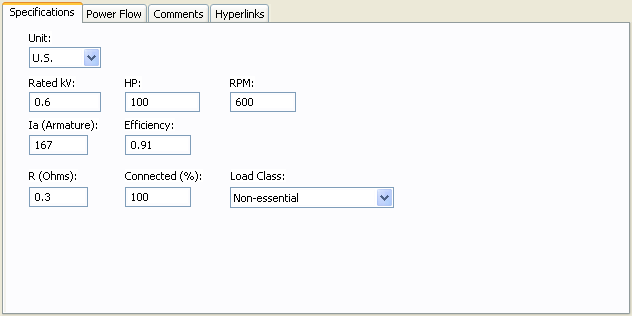Figure 3: Specifications Tab for DC Motors

Option Description
Unit Choose either US or Metric.
Rated kV Motor rated kV.
Hp/kW Motor rated horsepower (U.S. units) or kilowatts (metric units).
RPM Revolutions per minute.
Ia (Armature) Rated armature current in amps.
Efficiency Ratio of output mechanical power to input power.

R (Ohms)

Armature resistance in ohms. This affects the short circuit contribution.
Connected (%) Scales the short circuit contribution from the motor.
Load Class To specify the class in terms of importance. You can select Essential, Critical, or Non-essential. This field does not affect analysis. It can be used in database query to distinguish a certain load class from others. See Advanced Query for more information.

## Short Circuit Tab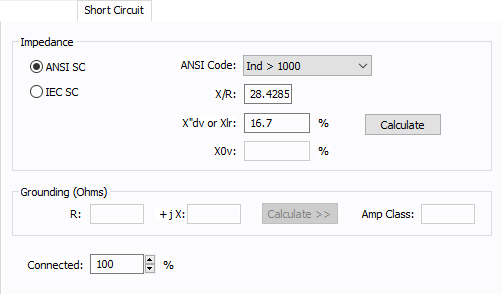Figure 4: Short Circuit Tab of Motor Data Dialog Box

Option Description
ANSI SC

Provides an easy way to enter ANSI Standard impedances and interrupting duty multipliers. Code numbers are chosen according to the motor types, sizes and modeling method. Regardless of the code chosen, ANSI Standard interrupting value multipliers are used.

The codes available in this list change depending on what you have selected in the Type field of the Specifications tab. Using the ANSI Code field is the recommended method to enter motor impedances to assure that the proper interrupting duty impedance multiplier is used for ANSI Standard calculations1,2,3,4.

If the Adjustable Frequency Drive check box is selected in the Specifications tab, the following choices are available for the ANSI Code field:

• Non-Regenerative: Motor does not contribute short circuit currents to upstream faults.
• Regenerative: Motor contributes to upstream faults. The impedance multipliers are used as per Conrad St. Pierre’s A Practical Guide to Short –Circuit Calculations.

Grouped motors with an adjustable frequency drive are non-regenerative motors and do not have contribution regardless of the motor type.

Reference:
1 AC High Voltage Circuit Breakers Rated on a Symmetrical Current Basis, ANSI/IEEE Std. C37.010-1979
2 Calculation of Fault Current for Application of AC High Voltage Circuit Breakers Rated on a Total Current Basis, ANSI/IEEE St. C37.5-1979
3 Low Voltage Power Circuit Breakers Used in Enclosures, ANSI/IEEE Std. C37.13-1981
4 Calculation of Short Circuit Currents with Contributions From Induction Motors, Walter C. Huening Jr., IEEE/IAS Mar/Apr 1982

IEC SC

When this option is selected, IEC short circuit calculations are used. See IEC Impedance and X/R Calculations.

If the motor has an adjustable frequency drive, you can also select the option for a regenerative or non-regenerative drive.

Grouped motors with an adjustable frequency drive are non-regenerative motors and do not have contribution regardless of the motor type.

X/R You can either type in the reactance to resistance ratio, or click Calculate to have EasyPower fill in this field.
X"d or Xlr

Subtransient reactance in percent on the motor Hp base. Normally this is 16.7% for induction motors.

If you have the locked rotor multiple (LRM) which is the ratio of the lock rotor current to the rated motor current, then you can calculate the impedance in percent using 100 / (LRM).

X0v

Saturated zero sequence reactance in percent on the motor’s MVA base. Zero sequence values are used in ground fault calculations.

Calculate Click to have EasyPower fill the X/R and X" or Xlr fields. You can specify the average HP for grouped motors in Tools > Options > Equipment.

Grounding

Grounding impedances only apply to wye grounded connections. The units are R+jX in ohms. If you only know the ground amperes of the circuit, enter the amp class and use the Calculate button to calculate the grounding impedance.

R

Neutral ground resistance in ohms. Grounding resistors are usually given in amperes. The impedance is found from the following equation:

R = Vln / I

Only wye grounded motors (YG) are modeled with grounds.

jX

Neutral ground reactance in ohms.

Calculate

Click to have EasyPower fill in the R and jX fields (if the Amp Class is specified) or to fill in the Amp Class field (if R and jX are specified).

Amp Class

This is the current in amps through the ground impedance at the rated voltage. You can enter data in this field directly in amps or calculate it based on the voltage and ground impedance R +jX using Calculate.

Connected

Provides an easy way to adjust the total motor horsepower used in determining short circuit currents. By changing the percent connected, the actual Hp (total connected value) entered in the Hp field can remain static. This reduces modeling errors and eliminates multiple databases for different contingencies.

For example, an MCC has a group of induction motors (all over 50 HP) that add up to a total load of 600 HP. However, 300 HP is considered backup and is not on-line. In order to keep proper records of the MCC HP, 600 would be entered in the HP field. Since only 300 HP is spinning at any one time and can contribute short circuit current, the connected field is set to 50%.

RMf / X"d

The ratio of fictitious resistance to the subtransient reactance that is used in peak current calculations. If left blank, EasyPower calculates the value based on the IEC-60909 standard.

This option appears for synchronous motors if the Show fields and X/R calculations based on IEC-60909 option is selected in Tools > Options > Equipment.

### ANSI Code Impedances

Impedance Multipliers for ANSI Std. Short Circuit Calculations

Code

Motor

Momentary Duty
First Cycle

Interrupting Duty
1.5 - 4 Cycles

Synch

Synchronous

1.0 X"dv

1.5 X"dv

Ind>1000

Induction Motor > 1000 Hp

1.0 X"dv

1.5 X"dv

> 250 @ 3600

Induction Motor > 250 Hp at 3600 RPM

1.0 X"dv

1.5 X"dv

>50

Induction Motor or Motor Group > 50 Hp

1.2 X"dv

3.0 X"dv

<50

Induction Motor Group < 50 Hp

1.67 X"dv

Infinite X"dv

Lumped

Lumped Induction Motor Group

1.0 X"dv (X=25%)

3.0 X"dv

Note: X" for induction motor groups >50 HP and <50 HP are typically assumed equal to 16.7%. Using the impedance multipliers, this corresponds to an equivalent motor contribution of 3.6 to 4.8 times the full load current.

## IEC Impedance and X/R Calculations

Model

Motor Type

Impedance and X/R Calculations

Individual

Induction without AFD

1) Calculate the MW per pole-pair by dividing MW by pole-pairs.

• MW is the rated output MW for the motor.
• Metric: MW = rated kW for motor / 1000.
• U.S: MW = HP * 0.746 / 1000.
• Pole-pairs = system frequency * 60 / motor sync RPM (from the Specifications tab.)

2) Calculate the X/R ratio of the motor.

• If the motor kV is greater than 1.0 and the MW per pole-pair is greater than or equal to 1.0, then X/R is 10.0.
• If the motor kV is greater than 1.0 and the MW per pole-pair is less than 1.0, then X/R is 6.667.
• If the motor kV is less than or equal to 1.0, the X/R ratio is based on the ANSI calculations.

Impedance is 16.7%.

Group

Induction without AFD

Impedance and X/R are calculated as follows:

• If motor kV less than or equal to 1.0, then impedance is 20% and the X/R ratio is 2.381.
• If the motor kV is greater than 1.0 and MW per pole-pair is greater than or equal to 1.0, then impedance is 16.7% the X/R ratio is 10.0.
• If the motor kV is greater than 1.0 and the MW per pole pair is less than 1.0, the X/R ratio is 6.667.

Individual or Group

Induction with AFD

DC with AFD

Impedance is 16.7% and the X/R ratio is 10.

Individual or Group

Synchronous

Synchronous Condenser

Uses the ANSI default calculations for impedance and the X/R ratio.

## TCC Tab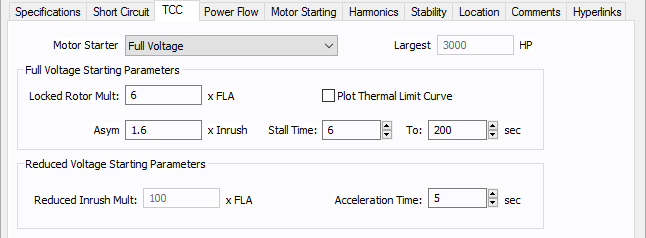Figure 5: TCC tab of Motor Data Dialog Box

Option Description
Motor Starter Type

Provides a list of motor starters. Select the appropriate starter from the following:

• Full Voltage: The motor starts at the rated voltage, without any starting device connected to it.
• Auto-transformer: An auto-transformer is used as a starting device to reduce the starting current. The tap value of the auto-transformer, as a percentage of the rated motor voltage, is entered in the edit field Auto-Xfrmr Tap at the bottom of the dialog box.
• Part-Winding: Part-winding start motors, in which only a part of the winding is used for starting. The entire winding is energized after start up. The Winding Tap must be specified at the bottom of the dialog box.
• Wye-Delta: The starter keeps the motor windings in wye-connection while starting to reduce the starting current. After start up the starter connects the windings in delta configuration.
• Reduced Voltage: The starting voltage during starting is less than the rated voltage. The inrush current for reduced voltage starting, as a multiple of full load amps, must be specified at the bottom of the dialog box.
Largest In grouped motor data, you can specify the size of the largest motor. This field is unavailable when you select the motor as individual motor. When you plot the motor starting curve on a time-current curve (TCC), the program plots the curve such that the largest motor is being started and the rest of the motors in the group are running at full load.

Full Voltage Starting Parameters

This section specifies the motor current characteristics during starting at the rated motor voltage.

Locked Rotor Multiple Locked rotor current as multiples of full load amps.
Asymmetrical (Asym) Offset Factor by which the inrush current is higher due to the asymmetry current.
Plot Thermal Limit Curve Check box to plot thermal limit time-current curve of the motor. The curve is shown within the limits of the Stall Time described below. The curve is an I2t curve passing through the Stall Time and the locked rotor amps.
Stall Time The maximum time in seconds that the motor can safely withstand stalling.
To The maximum time in seconds of the thermal limit curve of the motor you want to plot.
Reduced Voltage Starting Parameters

Specifies the starting time and the current or voltage ratio under reduced voltage. Depending upon the motor starter type selected enter one of the following. The starting current will be calculated from this.

• Auto-Xfrmr Tap: The auto-transformer tap ratio as a percentage of rated voltage.
• Winding Tap: Starter winding ratio as a percentage of full motor winding.
• Reduced Inrush Mult: The inrush current at reduced voltage, as a multiple of full load amps.
Acceleration Time The starting time in seconds.

## Power Flow Tab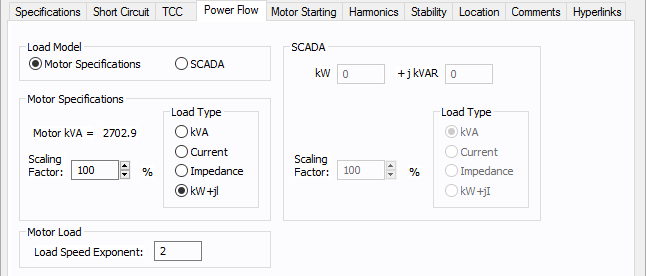Figure 6: Power Flow Tab of Motor Data Dialog Box

Option Description
Load Model Enables you to select the motor kVA from the Specified data or from SCADA (Supervisory Control and Data Acquisition) data. SCADA data can be read in by clicking File > Import.
Motor Specifications
Motor kVA Calculated from motor specifications. This is for reference only and cannot be changed except by new specifications.

Motors can be modeled for the power flow solution in several different ways.

• Constant kVA - This is the most common model. It is conservative, and results in slightly lower voltage values than would be measured on an actual system.
• Constant Current - This model is generally not used in motor modeling. It more closely matches an induction motor's characteristics in the reactive component than other models, but is technically incorrect because kW is relatively constant throughout the voltage range for an induction motor.
• Constant Impedance - This model is used for starting induction and synchronous machines, and closely matches motor characteristics during low voltages.
• kW + j Current - This model is a combination of the above models and more closely matches actual motor characteristics within normal operating voltages.
Scaling Factor Provides an easy way of adjusting the total motor load used in determining power flows. By changing the scaling factor, the actual Hp (total connected value) entered in the Hp field can remain static. This reduces modeling errors and eliminates multiple databases for different contingencies.

SCADA data is derived from real time, or metered data, and converted to an ASCII format which can be read into EasyPower. SCADA data is read in as a 100% scaling factor load. The load value is multiplied by the user-defined scaling factor. This provides a way to adjust SCADA loads to form new cases.

kW The kW value as read in from the SCADA ASCII file.
kVAR The kVAR value as read in from the SCADA ASCII file.

SCADA data can be modeled in the power flow solution in several different ways. SCADA load type is set in the ASCII file, but you can change it.

• Constant kVA - This is the most common model. It is conservative, and results in slightly lower voltage values than would be measured on an actual system.
• Constant Current - This model is generally not used in motor modeling. It more closely matches an induction motor's characteristics in the reactive component than other models, but is technically incorrect because kW is relatively constant throughout the voltage range for an induction motor.
• Constant Impedance - This model is used for starting induction and synchronous machines, and closely matches motor characteristics during low voltages.
• kW + j Current - This model is a combination of the above models and more closely matches actual motor characteristics within normal operating voltages.
Scaling Factor Provides an easy way of adjusting the total SCADA load used in determining power flows. By changing the scaling factor, the actual kW +j kVAR read in from the ASCII file remains static; however, the load used in the power flow is adjusted by this factor.

When you run power flow calculations, the motor load is scaled based on the frequency of the AFD. Speed is proportional to frequency. The simplified load model used is:

Output Power = (Rated Power) * (AFD frequency / System Frequency) ^ x.

Where x is the Load Speed Exponent.

If you have a machine in which the load (power) is independent of the speed, then set the exponent to 0. If machine load (power) is proportional to speed, then set the exponent to 1. Pump loads are closer to speed squared model, so set the exponent to 2. This is the default.

Example:

A 100HP motor rated at 60Hz is fed by AFD at 30Hz. If the Load Speed Exponent is 2, the power output at 30Hz would be:

100 * ( 30 / 60 ) ^ 2 = 25HP.

## DC Motor Power Flow Tab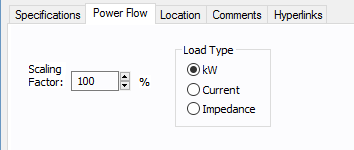Figure 7: DC Motor Data - Power Flow

Option Description
Scaling Factor Ratio of actual load to connected (rated) load in percent. This is used in power flow calculations.

Choose one of the following models:

• kW: The kW consumed remains constant even when terminal voltage changes.
• Current: Constant current is drawn by the motor even when terminal voltage changes.
• Impedance: The equivalent resistance for steady state is constant, so the kW and current will vary based on the terminal voltage.

## Motor Starting Tab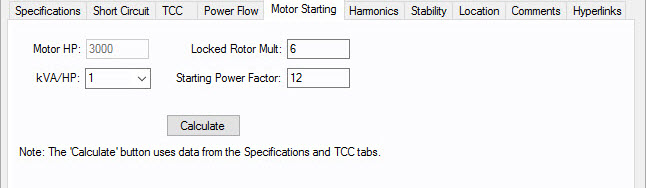Option Description
Motor kW The required input or rated power of the motor.
kVA/kW or kVA/HP Represents the efficiency of the motor. It is a conversion factor for delivered power and the required input power.
Locked Rotor Mult Equal to the inverse of the subtransient reactance (also known as X"dv) and provides the value at which the full load amp rating of the motor is multiplied under short circuit conditions.
Starting Power Factor The momentary power factor during the start of the motor. While the motor is under starting conditions, the reactive power is higher than the real power as a result of the starting power factor.
Calculate

Calculate uses data from the Specifications and TCC tabs to determine motor starting values. EasyPower references IEEE standards for the calculations.

For example, if Motor Starter on the TCC tab is set to something other than Full Voltage, it affects the calculations.

## Harmonics Tab

Use the Harmonics tab to indicate whether this equipment item is introducing harmonics into your power system.

###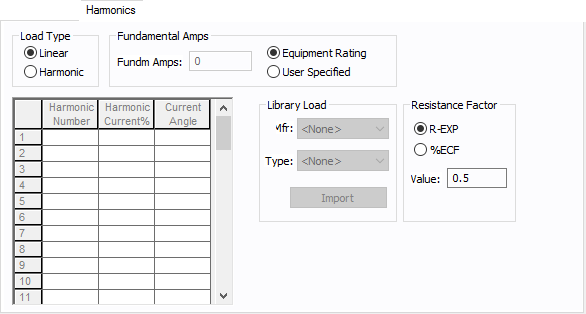Figure 8: Harmonics Tab

Option Description

The default is Linear, indicating the equipment does not produce harmonics. Choosing Harmonic makes the item an harmonic source and makes other fields in this tab available to edit.

Note: For an adjustable frequency drive (AFD), the Load Type is always Harmonic.
For motors, the Load Type is Harmonic if the With Adjustable Frequency Drive (AFD) check box is selected on the Specifications tab of the motor; otherwise, it is always Linear.

Fundamental Amps

Use to set the fundamental amps. The options are as follows:

• Equipment Rating sets Fundm Amps to the equipment rating of the item described in the Specifications tab.
• User Specified activates the Fundm Amps field, enabling you to specify a value.

To use fundamental current calculated by power flow, select Calculated from Power Flow in the Summation Fundamental Voltage area of the Harmonics Options > Control dialog box.

Use the spreadsheet to enter the harmonic spectrum produced by this item. You can enter up to 30 different harmonics in each equipment item. In the spreadsheet, enter the Harmonic Number (such as 5 for the 5th harmonic), the Harmonic Current in percent of the Fundamental Amps, and the Current Angle. By indicating the current angle, you can simulate transformer phase shift effects on rectifiers so appropriate canceling can take place. The harmonic may be integer or non-integer.

Common harmonic spectra may be entered from the device library. For instructions on how to enter your own spectra information, see Harmonics with Spectrum™. After selecting a particular device library spectrum from the Mfr and Type lists, click Import, and that spectrum is entered into the harmonic spreadsheet.

Resistance Factor

EasyPower offers two methods for calculating RH:

• Resistance varying with a power of the harmonic (R-EXP):
• RH = RFund * H R-EXP

• Resistance varying with a percent eddy current factor (%ECF):
• RH = RFund * (1+ECF*H2)/(1+ECF)

EasyPower defaults all skin effect correction to R-EXP and a value of 0.5.

Typical Resistance Correction Factors

R-EXP %ECF

Transformer

0.5-1.0

1.0-3.0

Utility

0.0-0.8

-

Generator

0.3-0.6

-

Line/Cable

0.5

-

Reactor

0.5-1.0

0.8-3.0

Motor

0.2-0.4

-

## Stability Tab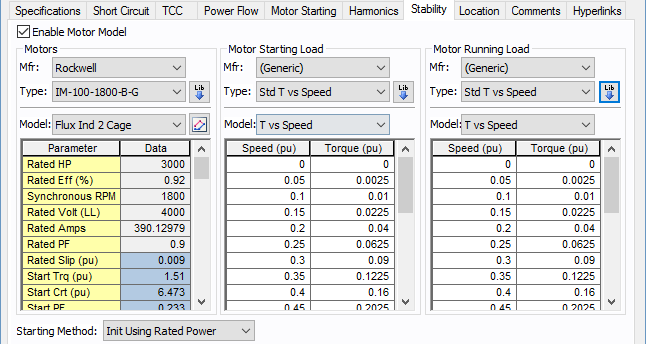Figure 9: Stability tab of Motor Data Dialog Box

Option Description
Enable Motor Model

Select the check box to enter stability information.

Without this, you cannot run a dynamic simulation for the motor.

Motors
Mfr Provides a list of manufacturers available in the device library. If the desired manufacturer is not listed in the device library, you can add it to the library.
Type Equipment types available from the selected manufacturer. If the desired type is not listed, you can add it to the library.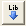Lib Populates the table with equipment data from the library. See EasyPower Device Library for more information.
Model Equipment models available from the selected equipment type. If the desired model is not listed, you can add it to the library.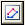Define Parameters Enables you to obtain an estimate of the motor circuit parameters from the torque vs. speed characteristics. See Define Motor Parameters (Step 1) .
Starting

This setting selects the method by which the motor will be initialized. The two options for this setting are:

• Init Using Rated Power: When initialized using rated power (default), the motor determines the slip necessary to maintain the terminal power conditions (kW) from the power flow case. Motor kVar is determined completely by the induction motor machine equations.
• Init Using Rated Slip: When initialized using rated slip, the motor forces terminal real power conditions (kW) to correspond to the specified rated slip of the motor. Thus, power conditions defined in the power flow case are abandoned to match slip. Motor kVar is determined completely by the induction motor machine equations.
• Explanation: There are conditions where a motor parameter derivation has significant errors in rated conditions (such as upwards to 10%) when attempting to match a manufacturer's torque vs. speed curve. This is most likely created by inconsistencies in the supplied data for various reasons. For such conditions, initializing to rated power defined by the power flow causes a new slip to manifest through initialization, which is not equal to the specified rated slip. This then creates a condition where the torque produced by the motor during starting can be significantly greater than the torque vs. speed curve generated in the parameter derivation. For a motor in a borderline start condition (near stalling), the results may show an incorrect successful start (we have seen up to 12% greater torque over the motor's speed range). To correct for this, select "Init Using Rated Slip". This will force the motor to re-create the exact torque vs. speed curve generated in the parameter derivation. Note however that the rated conditions reached after starting will have the error accepted in the parameter derivation.

This section defines the model for load characteristics while the motor is starting.

Mfr Provides a list of starting load characteristics available in the device library.
Type Starting load types available from the manufacturer selected in the Mfr field above.
Model

Lists available starting load models in the library. The choices are:

• Speed Squared: The torque is proportional to the square of the speed.
• Speed Cubed: The torque is proportional to the cube of the speed.
• Torque vs. Speed: User-defined spreadsheet.Lib Populates the table with equipment data from the library. See EasyPower Device Library for more information.

This section defines the model for load characteristics while the motor is running.

Mfr Provides a list of manufacturers available in the device library. If the desired manufacturer is not listed in the device library, you can add it to the library.
Type Equipment types available from the selected manufacturer. If the desired type is not listed, you can add it to the library.
Model Equipment models available from the selected equipment type. If the desired model is not listed, you can add it to the library.Lib

Populates the table with equipment data from the library. See EasyPower Device Library for more information.

You can also use the Define Motor Parameters feature described below to derive the motor parameters.

### Define Motor Parameters (Step 1)

ClickDefine Motor Parameters to obtain an estimate of the motor circuit parameters using the name-plate values and the speed-torque curve of the motor. Notes provided in the upper left corner of this window are instructions on how to use this tool to derive the motor parameters.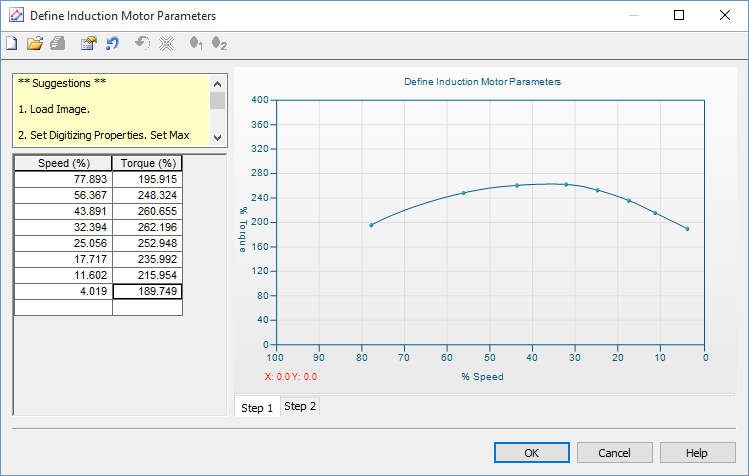Figure 10: Define Induction Motor Parameters-Step 1

Option Description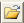Load image Enables you to load an image of the motor curve to be digitized.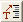Set max torque Enter motor maximum torque in %.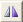Flip speed axis Curve axis can be flipped to match the manufacturer.

### Define Induction Motor Parameters (Step 2)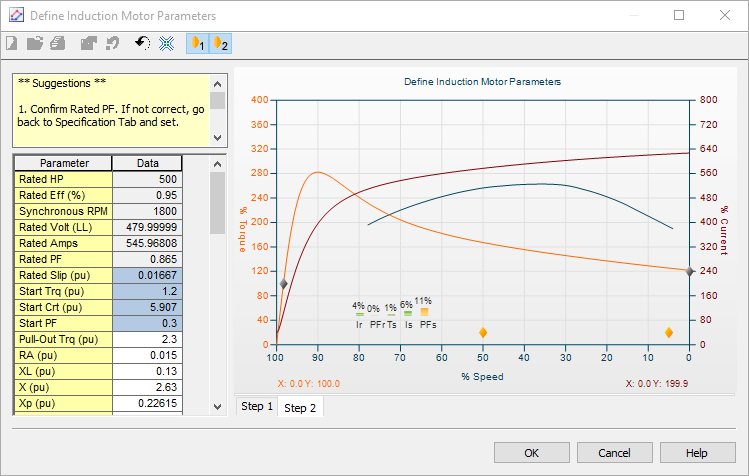Figure 11: Define Induction Motor Parameters-Step 2

Option Description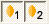Gravity Points

The gravity points are movable points used to optimize the derived torque speed curve to the actual torque speed curve.Flip speed axis

Curve axis can be flipped to match manufacturer.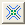Minimize

The Minimize button attempts to reduce the error.

## Other Tabs

See Common Tabs for information on the Location, Comments, Hyperlinks, or Collected Data tabs.

 Database Technical Reference Common Tabs Media Gallery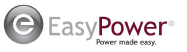EasyPower LLC

7730 SW Mohawk St.

Tualatin, OR 97062

(503)655-5059

EasyPower Version 10.2